### Linear Elastic Materials: Restrictions on the Elastic Moduli

#### Learning Outcomes

Describe the mathematical concept: “positive definiteness” and its relationship to the physics concept “energy storage” in the material.
State the limits on Young’s modulus and Poisson’s ratio for linear elastic isotropic materials for the material to be stable (with a positive definite relationship between the stress and the strain).
Compute the energy stored inside a material for the simple cases of: “shear stress”, “hydrostatic stress”, and “uniaxial stress”.

### Restrictions on the Elastic Moduli

The strain energy density per unit volume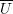for a linear elastic material under a general state of stress is equal to: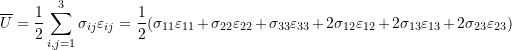As shown above, since each of the stress and strain matrices are symmetric, they can be represented as vectors in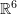. The dot product can then be used to find a simplified expression for the strain energy density function: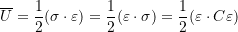We can use two physical restrictions on the materials to impose mathematical restrictions on the entries of the matrix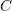. These two restrictions are that the material is stable and deformable. Stability means that whenever a state of strain is applied, energy is stored in the material. Stability is also equivalent to the statement that the material deformation increases in the direction of the increasing stress. The equivalent mathematical restriction onis: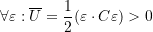Sinceis symmetric, thenhas to be positive definite. This implies that all its eigenvalues have to be positive. This argument will be used to find restrictions on the values of the elastic moduli described above.

#### Restrictions on the Elastic Moduli of Linear Elastic Isotropic Materials

The distinct eigenvalues of the matrixin Equation 6 are: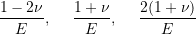For these to be positive, the following inequalities have to be satisfied: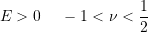The following mathematica code presents the calculations above:

View Mathematica Code

G = Ee/2/(1 + Nu);
Cc = {{1/Ee, -Nu/Ee, -Nu/Ee, 0, 0, 0}, {-Nu/Ee, 1/Ee, -Nu/Ee, 0, 0,0}, {-Nu/Ee, -Nu/Ee, 1/Ee, 0, 0, 0}, {0, 0, 0, 1/G, 0, 0}, {0, 0,0, 0, 1/G, 0},
{0, 0, 0, 0, 0, 1/G}};
Cc // MatrixForm
a = Eigenvalues[Cc]
Reduce[{a[]>0, a[]>0,a[]>0}, {Ee, Nu}]

View Python Code

from sympy import Matrix,fraction
from sympy.solvers.inequalities import reduce_rational_inequalities
import sympy as sp
Ee,Nu = sp.symbols("E nu", real = True)
G = Ee/2/(1 + Nu)
Cc = Matrix([[1/Ee,-Nu/Ee,-Nu/Ee,0,0,0],
[-Nu/Ee,1/Ee, -Nu/Ee,0,0,0],
[-Nu/Ee, -Nu/Ee, 1/Ee,0, 0, 0],
[0, 0, 0, 1/G, 0, 0],
[0, 0, 0, 0, 1/G, 0],
[0, 0, 0, 0, 0, 1/G]])
display("C =",Cc)
eigensystem = Cc.eigenvects()
eigenvalues = [i for i in eigensystem]
display("eigenvalues =",eigenvalues)
# I have not found an algorithm where sympy can solve multiple values in inequalities
n1,d1 = fraction(eigensystem)
n2,d2 = fraction(eigensystem)
n3,d3 = fraction(eigensystem)
s_Nu = reduce_rational_inequalities([[n1>0,n2>0,n3>0]], Nu)
s_Ee = reduce_rational_inequalities([[1/d1>0,1/d2>0,1/d3>0]], Ee)
display("Inequalities for Eigenvalues to be positive:",s_Nu,s_Ee)


Materials possessing a negative Poisson’s ratio are common, see for example the article here or the Wikipedia article on Auxetics (materials possessing negative Poisson’s ratio).

A more intuitive method to find the same restrictions is by assuming different states of stresses and ensuring that the energy stored per unit volume under these states of stresses is positive. The first state is a uniaxial state of stress where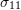is the only non-zero stress component. Using Equation 6, the stresses and the strains have the following forms: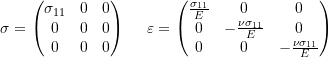The restriction that the energy stored has to be greater than zero leads to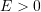as follows: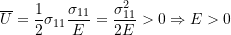The second state is a shear state of stress where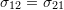are the only non-zero stress components. Using Equation 6, the stresses and the strains have the following forms: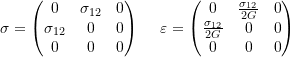The restriction that the energy stored has to be greater than zero leads to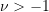as follows: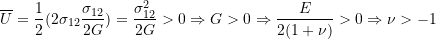The third state is a state of hydrostatic stress where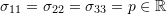are the only non-zero stress components. Using Equation 6, the stresses and the strains have the following forms: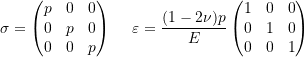The restriction that the energy stored has to be greater than zero leads to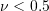as follows: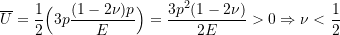#### Restrictions on the Elastic Moduli of Linear Elastic Orthotropic Materials

For orthotropic linear elastic materials, the positive definiteness ofimply the following inequalities: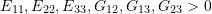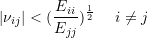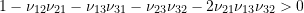The above equations show that values for the different orthotropic Poisson’s ratios are not bounded by –1 and 0.5. In fact, orthotropic materials (typically, fibrous biological materials like ligaments) can exhibit values beyond those bounds. For example, ligaments can exhibit a Poisson’s ratio that is higher than 0.5.

##### IMPORTANT NOTE:

The above restrictions ensure that, physically, a material is stable (possessing a rising stress strain curve) and that any state of stress will cause nonzero strain values inside the material. It is important to realize that it cannot be argued that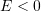and/or that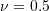are physically impossible. They are simply unacceptable constants for linear elastic materials with a positive definite constitutive model. Materials that become unstable with loading (e.g., decrease in stress accompanying an increase in strain) or incompressible materials (materials exhibiting no strain for a stress state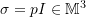,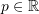may exist but cannot be modelled using the linear elastic constitutive model.